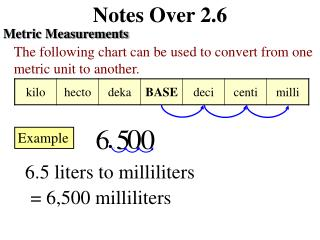DownloadDownload PresentationNotes Over 2.6

# Notes Over 2.6

Download Presentation## Notes Over 2.6

- - - - - - - - - - - - - - - - - - - - - - - - - - - E N D - - - - - - - - - - - - - - - - - - - - - - - - - - -
##### Presentation Transcript

1. Notes Over 2.6 Metric Measurements The following chart can be used to convert from one metric unit to another. Example 6.5 liters to milliliters = 6,500 milliliters

2. Notes Over 2.6 Metric Measurements The following chart can be used to convert from one metric unit to another. Example 70 centimeters to meters = 0.7 meters

3. Notes Over 2.6 Metric Measurements The following chart can be used to convert from one metric unit to another. 1. 25 meters to kilometers = 0.025 kilometers

4. Notes Over 2.6 Metric Measurements The following chart can be used to convert from one metric unit to another. 2. 7.9 milligrams to grams = 0.0079 kilometers

5. Notes Over 2.6 Metric Measurements The following chart can be used to convert from one metric unit to another. 3. 15 kiloliters to liters = 15,000 liters

6. Notes Over 2.6 Metric Measurements The following chart can be used to convert from one metric unit to another. 4. 6.5 decimeters to meters = 0.65 meters

7. Notes Over 2.6 Metric Measurements The following chart can be used to convert from one metric unit to another. 5. 450 millimeters to centimeters = 45 centimeters

8. Notes Over 2.6 Metric Measurements The following chart can be used to convert from one metric unit to another. 6. 0.09 kilometers to decimeters = 900 decimeters

9. Notes Over 2.6 Extra Practice Worksheet 2.6 Pg. 2-31, Lesson 2.6 #5-18 Worksheet 90/123